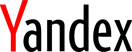(panoramic photo of Moscow from moscow.photobase.ru)# Eighth International Conference on Computability, Complexity and Randomness (CCR 2013)

### September 23–27, 2013, Moscow, Russia## Probabilistic algorithms in computability theory

Laurent Bienvenu
Can we compute with randomness something we cannot without? While complexity theorists still struggle with the fascinating question BPP =? P, the analogue problem in computability theory has seemingly been solved a long time ago: in 1954, de Leeuw, Moore, Shannon, and Shapiro proved that if a function can be computed by a probabilistic algorithm (= Turing machine), then it can be deterministically computed. However, the situation changes if one wishes to probabilistically generate \textbf{some} function in a class. For example, Martin showed that there exists a probabilistic algorithm which, with positive probability, computes some function which is not dominated by any computable one (obviously there is no way to computably and deterministically compute such a function). In fact, Martin's proof was quite abstract, but the best way to understand it is via an explicit probabilistic algorithm. I will present the proof of this result and several others classical theorems of the same type. I will then present some more recent work connecting the idea of probabilistic algorithm to reverse mathematics.